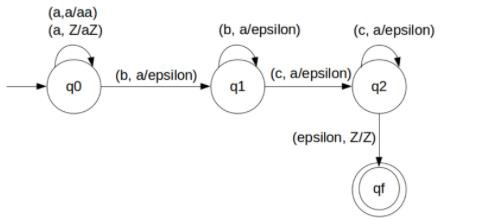# Construct DPDA for a(n+m)bmcn n,m‚â•1 in TOC

A push down automata (PDA) can be formally described as seven tuples

(Q, Σ,S, δ,q0,I,F)

Where,

• Q is finite number of states
• Σ is input alphabet
• S is stack symbol
• Δ is the transition function: QX(Σ∪{e})XSXQ
• q0 is the initial state (q0 belongs to Q)
• I is the initial state top symbol
• F is a set of accepting states

## Problem

Construct PDA for a(n+m)bmcn n,m≥1.

## Solution

So, the strings which are generated by the given language are −

L={aabc,aaaabccc,aaaaabbccc,….}

That is to add the number of b's and c's, and that will equal the number of a's.

For every b's and c's we will pop a's from the stack.

Let’s count the number of a's and that is equal to the sum of b's and c's.

It can be achieved by pushing a's in stack and then we will pop a's whenever "b" or"c" comes.

Finally, at the end of the strings if nothing is left in the STACK then we can say that language is accepted in the PDA.

The PDA for given problem is as follows −## Transition functions

The transition functions are as follows −

Step 1: δ(q0, a, Z) = (q0, aZ)

Step 2: δ(q0, a, a) = (q0, aa)

Step 3: δ(q0, b, a) = (q1, ε)

Step 4: δ(q1, b, a) = (q1, ε)

Step 5: δ(q1, c, a) = (q2, ε)

Step 6: δ(q2, c, a) = (q2, ε)

Step 7: δ(q2, ε, Z) = (qf, Z)

## Explanation

Step 1 − Let’s take an input string: "aaaabbcc" which satisfies the given condition.

Step 2 − Scan string from left to right.

Step 3 − For input 'a' and STACK alphabet Z, then

Push the input 'a' into STACK: (a,Z/aZ) and state will be q0

Step 4 − For input 'a' and STACK alphabet 'a', then

Push the input 'a' into STACK: (a,a/aa) and state will be q0

Step 5 − For input 'a' and STACK alphabet 'a', then

Push the input 'a' into STACK: (a,a/aa) and state will be q0

Step 6 − For input 'a' and STACK alphabet 'a', then

Push the input 'a' into STACK: (a,a/aa) and state will be q0

Step 7 − For input 'b' and STACK alphabet 'a' and state is q0, then

Pop one 'a': (c,a/ε) and state will be q1

Step 8 − For input 'b' and STACK alphabet 'a' and state is q1, then

Pop one 'a': (c,a/ε) and state remain q1

Step 9 − For input 'c' and STACK alphabet 'a' and state is q1, then

Pop one 'a': (c,a/ε) and state will be q2

Step 10 − For input 'c' and STACK alphabet 'a' and state is q2, then

Pop one 'a': (c,a/ε) and state will be remain q2

Step 11 − We reached end of the string, on input ε and STACK alphabet Z, then

Go to final state(qf): (ε, Z/Z)